# The converged application of EMQX + CNN in AIoT

2020-10-30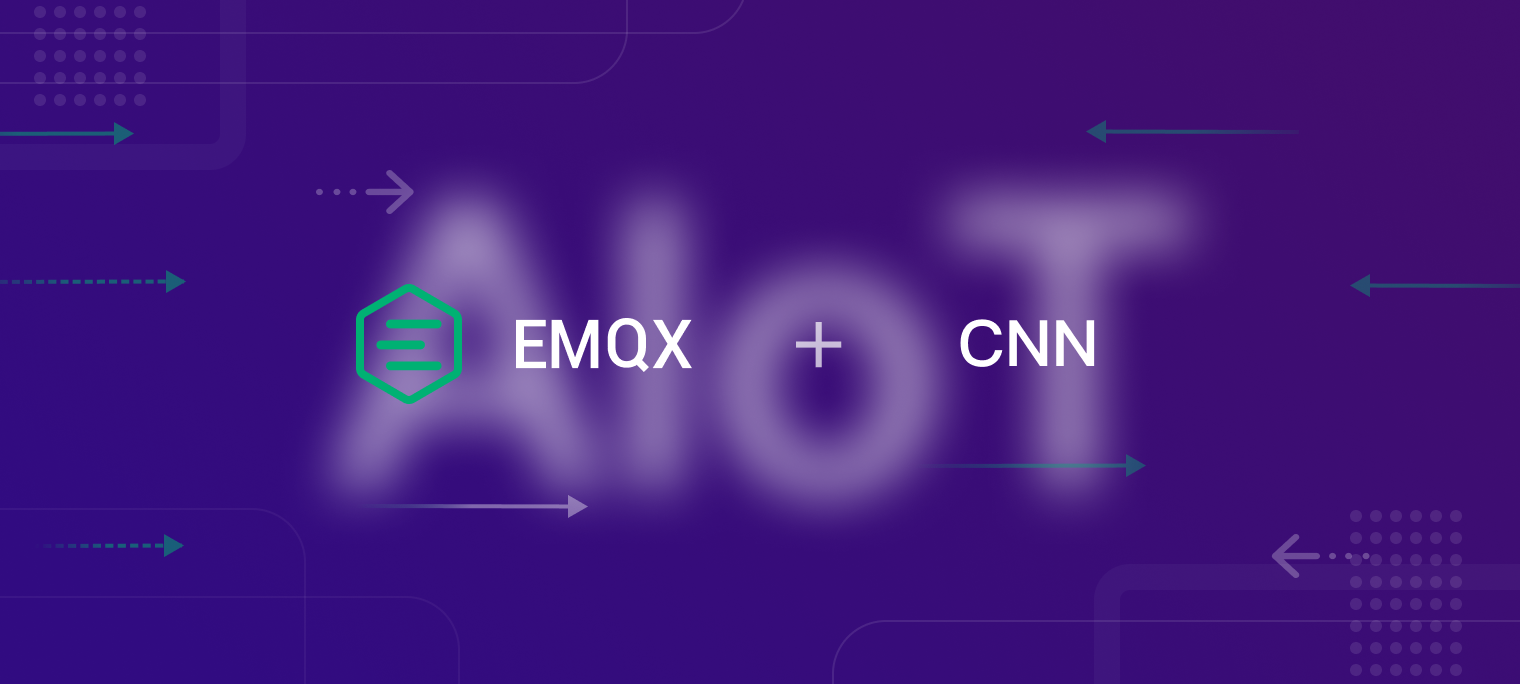People are familiar with IoT and AI. As the popular technology concept, they are both related to data: IoT solves where data comes from, while AI solves where data goes and what it is used for. The new concept 「AIoT」was also born that combines the two: IoT provides massive data for AI through connecting and communicating everything, and then AI will transfer these data into valid information through continuous learning and analyzing it.

In this article, we will present a simple fusion application of AIoT: use IoT message middleware EMQX Broker to collect hydraulic system temperature sensor data and forward it to a 1D Convolutional Neural Network. We will use this AI deep learning representative algorithm to predict the hydraulic system cooler state.

On a 1D Convolutional Neural Network, time will be viewed as a spatial latitude, and each output time step is obtained by using a small segment of the input sequence in the time dimension. Therefore, we can use this feature to implement the prediction of the time-series data. We will use Python code to simulate the temperature sensor time-series data, and transfer it to the EMQX Broker through MQTT protocol. Besides that, we will use its flexible rules engine to forward the data to a webhook, and will implement the state prediction of the hydraulic system cooler according to the input temperature sensor time-series data.

## Preparation of the data set

In this article, we will use the hydraulic system condition monitoring data set provided by the UCI machine learning and intelligent systems center. The data set was experimentally obtained with a hydraulic test rig. This test rig consists of a primary working and a secondary cooling-filtration circuit which are connected via the oil tank. The system cyclically repeats constant load cycles (duration 60 seconds) and measures process values such as pressures, volume flows and temperatures while the condition of four hydraulic components (cooler, valve, pump and accumulator) is quantitatively varied.

• In this data set, TS1.txt, TS2.txt, TS3.txt, TS4.txt are the temperature data from the four cooler temperature sensors of the hydraulic system with a cycle of 60 seconds, respectively.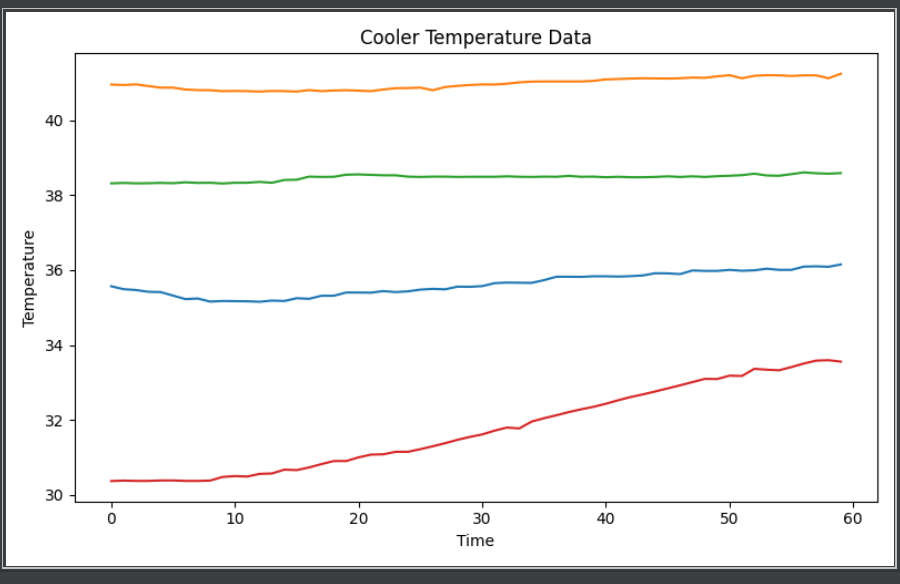• The first column of profile.txt indicates the state of the hydraulic system cooler during the current cycle.

• 3: close to total failure
• 20: reduced efficiency
• 100: full efficiency

## Model training

We will use a 1D Convolutional Neural Network to implement the model training. 1D CNN can be used to analyze the time-series of the temperature sensor data. In this article, we will use the sequential model described in this article to construct a 1D Convolutional Neural Network and will properly adjust the data set for improving the prediction accuracy.

• The model construction of the 1D Convolutional Neural Network

``````num_sensors = 4
TIME_PERIODS = 60
BATCH_SIZE = 16
EPOCHS = 10
model_m = Sequential()
print(model_m.summary())
history = model_m.fit(X_train, y_train, batch_size=BATCH_SIZE, epochs=EPOCHS, validation_split=0.2, verbose=2)
``````
• The report of indicators for classify model

From the report, we can see that the temperature data predicts the cooler condition with 95%, 80% and 89% accuracy for 3 (near failure), 20 (low efficiency) and 100 (full efficiency) respectively.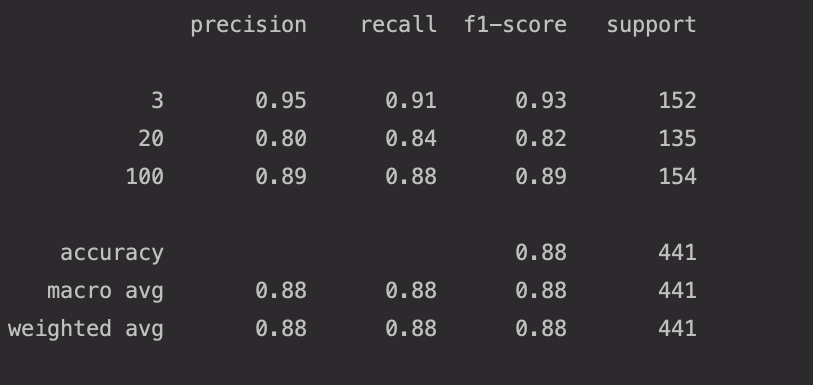## The simulation of data input

In this article, we will simulate reporting the cooler temperature sensor data in a production environment. Therefore, we will use Python code to read the temperature data in the dataset and report it to the EMQX Broker via the MQTT protocol.

In the following code, we firstly use `pandas` to read the temperature data in the dataset ('TS1.txt', 'TS2.txt', 'TS3.txt', 'TS4.txt'), and will simply process the data, and then report the data to the EMQX Broker every second.

``````import json
import time

import pandas as pd
from paho.mqtt import client as mqtt_client

broker = '127.0.0.1'
port = 1883
topic = "/1dcnn"
client_id = f'1dcnn-client'

def connect_mqtt():
def on_connect(client, userdata, flags, rc):
if rc == 0:
print("Connected to MQTT Broker!")
else:
print("Failed to connect, return code %d\n", rc)

client = mqtt_client.Client(client_id)
client.on_connect = on_connect
client.connect(broker, port)
return client

names = ['TS1.txt', 'TS2.txt', 'TS3.txt', 'TS4.txt']
df = pd.DataFrame()
for name in names:
data_file = f'./dataset/{name}'
df = df.sort_index()
df_values = df.values
df = df_values.reshape(-1, 4, len(df.columns))
data = df.transpose(0, 2, 1)
return data

def publish(client):
for x_data in data[-10:]:
for y_data in x_data:
t_1, t_2, t_3, t_4 = tuple(y_data)
msg = {
't1': round(t_1, 3),
't2': round(t_2, 3),
't3': round(t_3, 3),
't4': round(t_4, 3)
}
time.sleep(1)
result = client.publish(topic, json.dumps(msg))
# result: [0, 1]
status = result
if status == 0:
print(f"Send `{msg}` to topic `{topic}`")
else:
print(f"Failed to send message to topic {topic}")

def run():
client = connect_mqtt()
client.loop_start()
publish(client)

if __name__ == '__main__':
run()
``````

## Failure prediction

We will use the EMQX Broker rule engine to forward the data of temperature sensor to webhook, and will implement the prediction of the cooler state through the data collected by the temperature sensor.

1. Writing Webhook code

```python import asyncio import json

import numpy as np import uvicorn from keras.models import load_model from sklearn.preprocessing import StandardScaler from starlette.applications import Starlette from starlette.background import BackgroundTask from starlette.responses import JSONResponse

app = Starlette() queue = asyncio.Queue() model = load_model('./1d-cnn.h5')

@app.on_event('startup') async def on_startup(): print('startup webhook')

async def predictive(data): y_label = { 0: 3, 1: 20, 2: 100 } y_status = { 3: 'close to total failure', 20: 'reduced efficiency', 100: 'full efficiency' } x_test = np.array(data) scaler = StandardScaler() x_test = scaler.fit_transform(x_test.reshape(-1, x_test.shape[-1])).reshape(x_test.shape) x_test = x_test.reshape(-1, x_test.shape, x_test.shape) results = model.predict(x_test) msg = "Current cooler state probability: " for i, probability in enumerate(results): status = y_status[y_label[i]] msg += f"{probability * 100:.2f}% {status}({y_label[i]}), " print(msg)

def clear_queue(q): items = [] while not q.empty(): items.append(q.get_nowait()) return items

if name == 'main': uvicorn.run( app, host='127.0.0.1', port=8080, loop='uvloop', log_level='warning' )

``````
2. EMQX Broker resource creation

![WechatIMG2495.png](https://assets.emqx.com/images/81f1d87027ce3507ccdefd76ea8475a7.png)

3. EMQX Broker rule creation

![WechatIMG2496.png](https://assets.emqx.com/images/d06f7fa3985be14c0342a43f411c5a3e.png)

## Test

1. Enable Webhook

```bash
python3 webhook.py
``````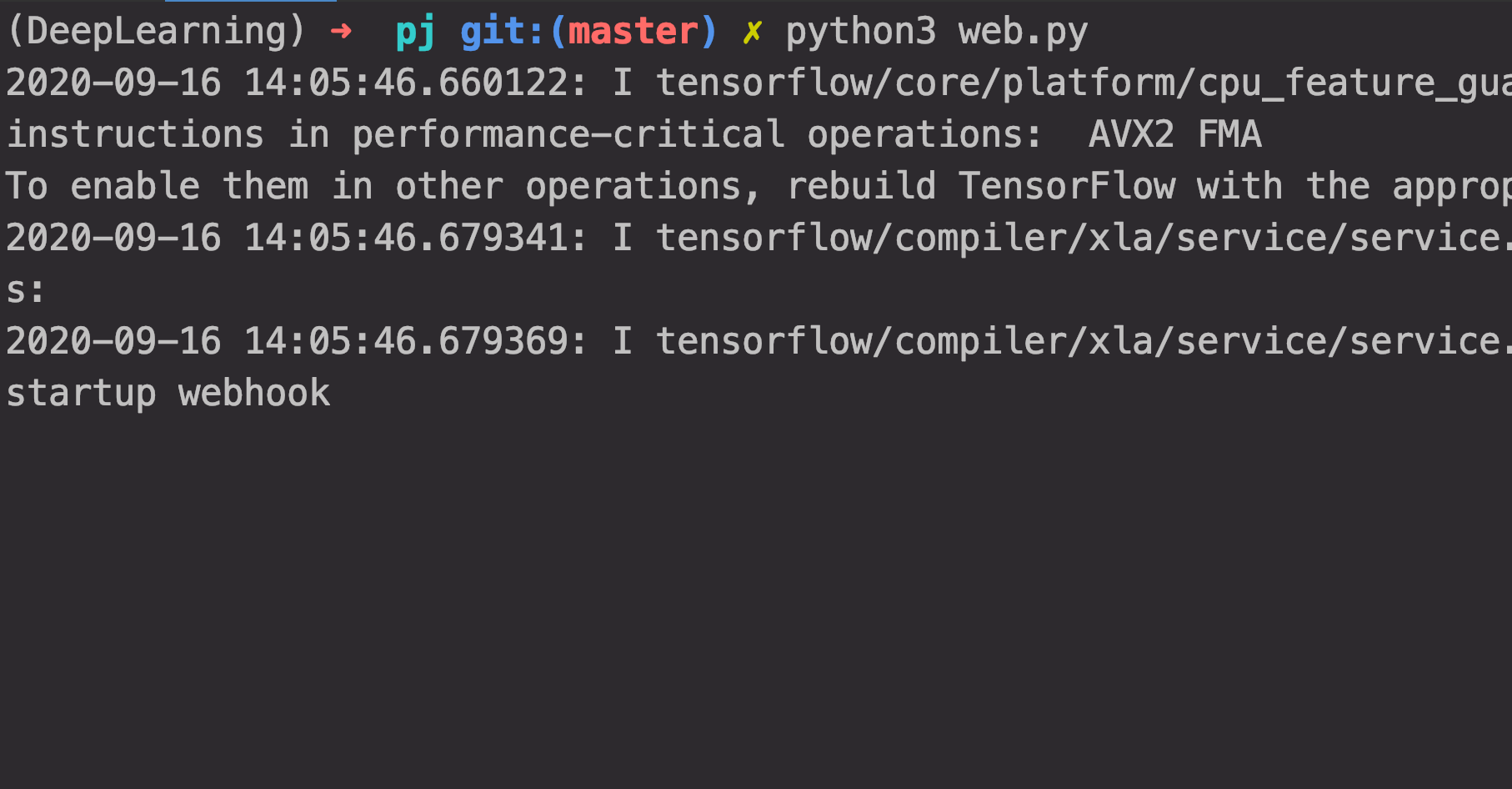1. Enable EMQX Broker

``````./bin/emqx start
``````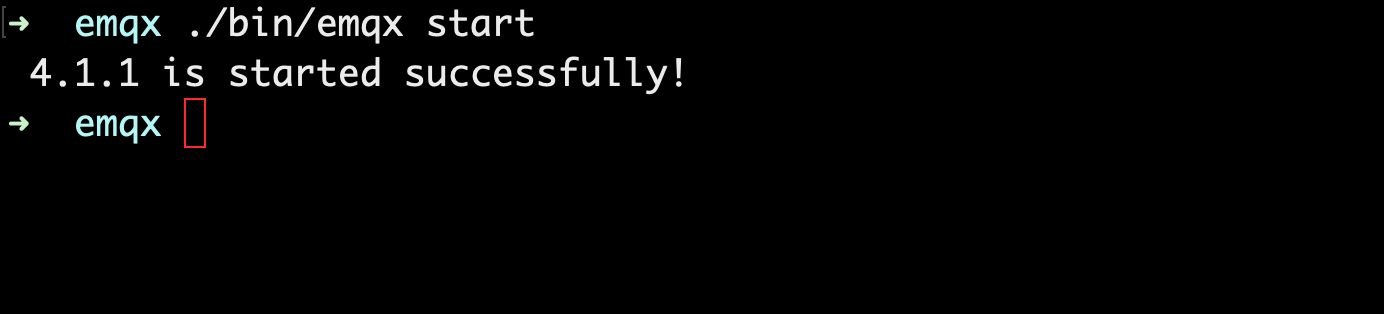2. Simulate data input

``````python publish.py
``````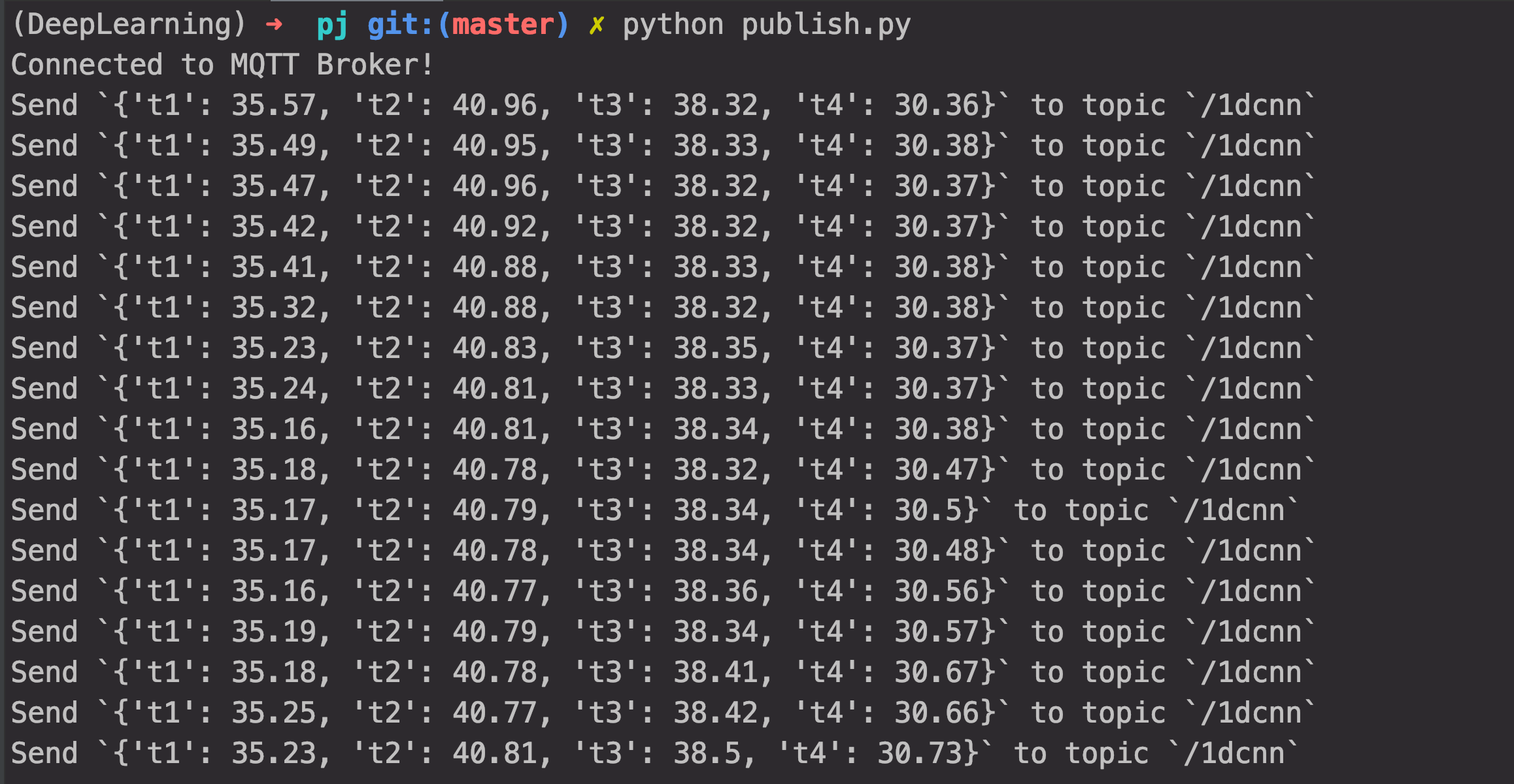3. View prediction results of the state of hydraulic system cooler

From the following picture, we can see that the current cooler state predicted by the input sensor temperature was close to total failure for the first five cycles, which is consistent with the cooler state given in the dataset.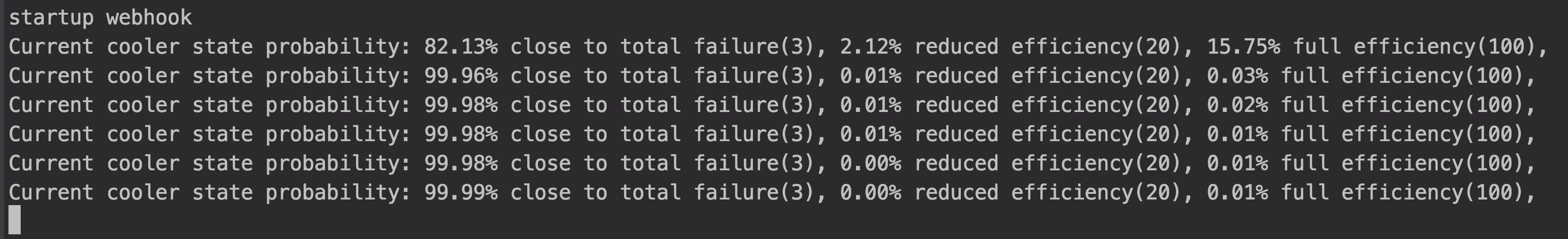4. Adjust the input data respectively, view the prediction result of the cooler state under different temperatures, and compare with the experiment result collected from the lab bench in the dataset.

• Input the data of the temperature sensor for the first ten cycles, view the prediction result, and compare with the experiment result collected from the lab bench.

Modify the file publish.py: for x_data in data: -> for x_data in data[:10]:From the above picture, we can see that the prediction result is consistent with the result collected from the lab bench.

• Select data in which the data set status is 3 (close to total failure) and 20 reduced efficiencies as inputs to view the predictions and compare them with the results collected from the lab bench.

Modify the file publish.py: for x_data in data: -> for x_data in data[728:737]: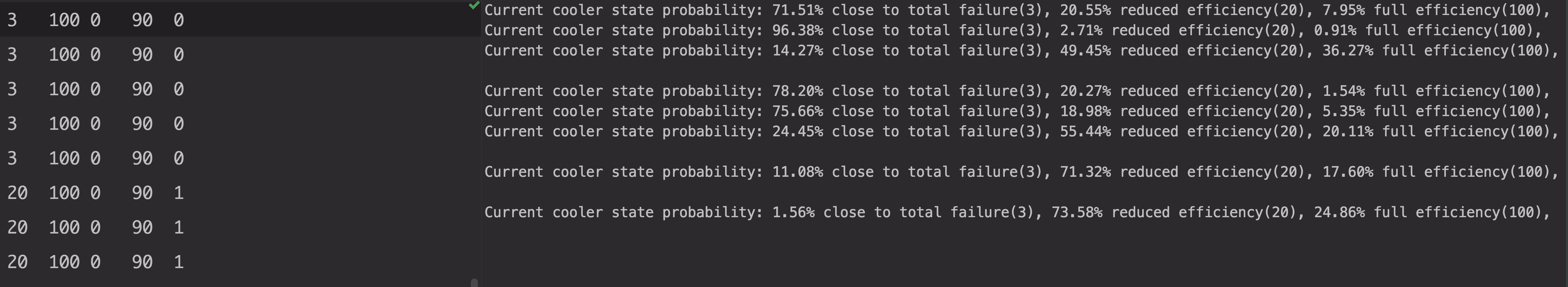From the picture above, we can see that there is some error between the predictions and the results collected from the lab bench, which validates the prediction accuracy probabilities reported in the model's classification indicators.

• Input the data of the temperature sensor for the last ten cycles, view the prediction result, and compare with the experiment result collected from the lab bench.

Modify the file publish.py: for x_data in data: -> for x_data in data[-10:]: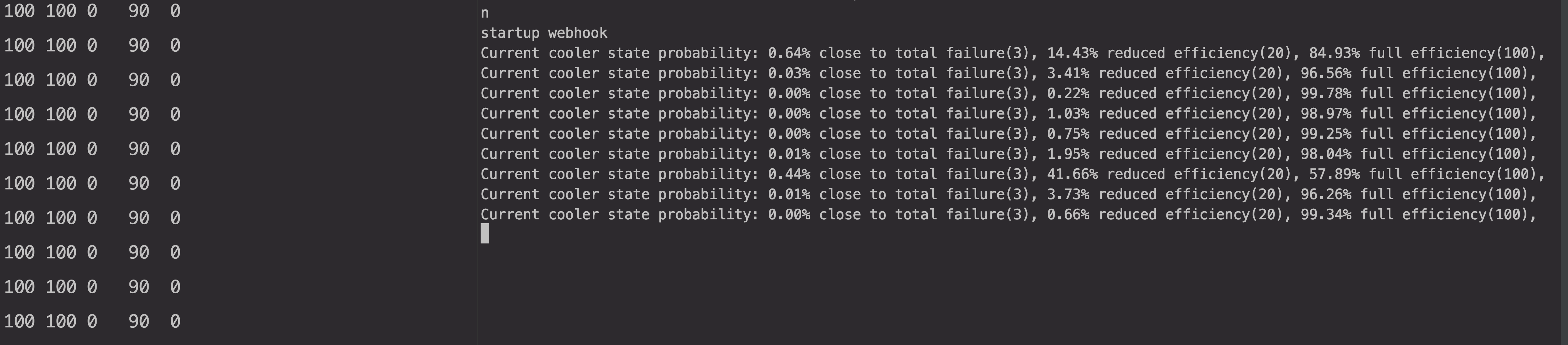From the picture above, we can see that the predictions are similar to the results collected from the lab bench, but there is still some deviation.

## Summary

So far, we have implemented sensor data reporting, data forwarding using the EMQX rule engine, and hydraulic system cooler fault prediction using a 1D Convolutional Neural Network.

In various fields of industry, whether it is machinery, electronics, iron and steel, or manufacturing, rubber, textile, chemical, food, hydraulic drive technology has become a basic application technology. With the continuous development of the modern industry, the hydraulic system becomes more high performance and high accuracy. Its reliability becomes more important, and the detection and diagnosis of hydraulic system faults is also more and more attention. Use AI and deep learning to monitor the status of the hydraulic system through IoT big data collection and analysis, which is for implementing the fault prediction. It is the new possibility brought by AIoT to the traditional industrial field.

In the actual application of hydraulic system failure prediction in various fields, to obtain a more accurate prediction using AI, it is necessary to collect a higher level of time-series data for analysis and training. Therefore, it is necessary to choose a highly stable and reliable messaging middleware with outstanding performance specifications to access and transmit large amounts of data. As an open-source MQTT messaging server with high concurrency and low latency, supporting distributed cluster architecture, EMQX Broker can meet the need for data transmission in this application scenario and other more IoT applications.